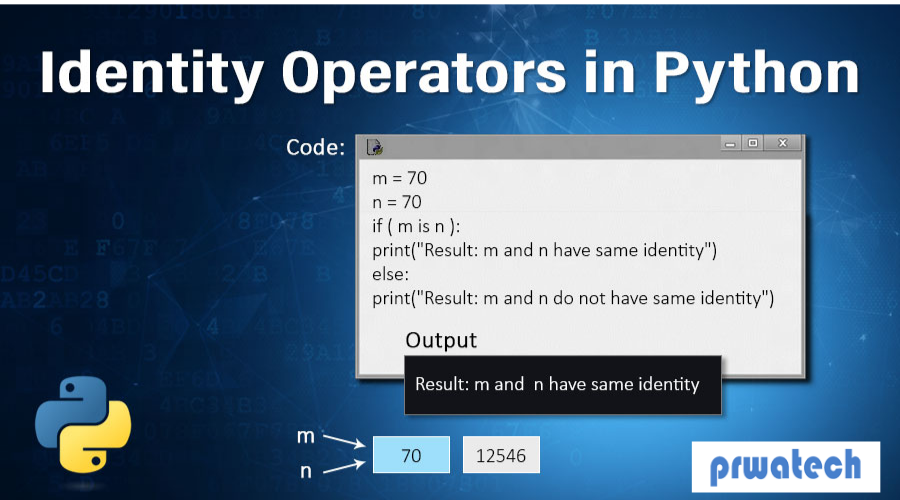### Python Operators

• date 17th July, 2019 |
• by Prwatech |

# Python Operators Tutorial

Python Operators Tutorial, In this tutorial, we will learn Operators in Python and how to use Operators in Python programming language. Here, You will also learn various types of Operators in Python programming language which are helpful for any Python developers. Are you looking for the information of Python Operators guide or Are you dreaming to become to certified Pro Python Developer, then stop just dreaming, get your Python certification course from India’s Leading Python training institute.

In Python Programming Language, there are a set of special symbols that perform various functions such as Arithmetic operators, Logical operators, Comparison operators, and many more which are known as Python Operators. There is a unique kind of Operations for every symbol or operator. Operands are the values on which the operators are used to perform their respective operations. Do you want to know the types of Python Operators and how to use operators in Python, then just follow the below mentioned Python Operators guide from Prwatech and take advanced Python training like a Pro from today itself under 10+ years of hands-on experienced professionals?

## Types of Operators in Python Programming Language

Operators in Python programming language are used to performing operations between two variables.

Python divides the operators into the following groups:

x=20

y=3

### Arithmetic operators in Python

 Operator Name Example Result + Addition x+y 23 - Subtraction x-y 17 * Multiplication x*y 60 / Divide x/y 6.66 % Module 20%3 2 ** Exponentiation 20**3 8000 // Floor division 20//3 6

### Assignment operators in Python

 Operator Example Result = x=y += x+=2 22 -= x-=2 18 *= x*=2 40 /= x/=2 10 %= x%=2 0 //= x//=3 6 **= x**3 8000 &= x&=0 False |= x|=0 True ^= x^=0 False >>= x>>=3 True <<= X<<=3 False

### Logical operators in Python

 Operator Description Example Result and Returns true if both variables are true x and 0 False or Returns true if either of the one variables is true x or 0 True not Returns opposite result of variable not(x or y) False

### Comparison operators in Python

 Operator Name Example Result == Equal x==20 True != Not Equal x!=y True > Greater Than x>y False < Less Than x= Greater Than Equal To x>=20 True <= Less Than Equal To x<=y False

### Identity operators in PythonOperator Description Example Result is Returns true if both variables are the same object x is y False is not Returns true if both variables are not the same object x is not y True

### Membership operators in Python

 Operator Description Example Result in Returns True if a sequence with the specified value is present in the object x in y False not in Returns True if a sequence with the specified value is not present in the object x  not in y True

### Bitwise operators in Python

 Operator Name Description & AND Sets each bit to 1 if both bits are 1 | OR Sets each bit to 1 if one of two bits is 1 ^ XOR Sets each bit to 1 if only one of two bits is 1 ~ NOR Inverts all the bits << Zero files left shift Shift left by pushing zeros in from the right and let the leftmost bits fall off >> Signed right shift Shift right by pushing copies of the leftmost bit in from the left, and let the rightmost bits fall off

Become a certified expert in Python Programming by getting enrolled from Prwatech E-learning India’s leading advanced Python training institute in Bangalore. Register now for more updates on Python Operators upgrades. Our expert trainers will help you towards mastering real-world skills in relation to these Python programming.

### Quick Support